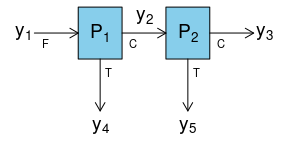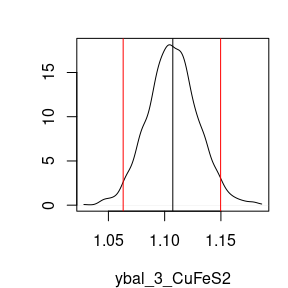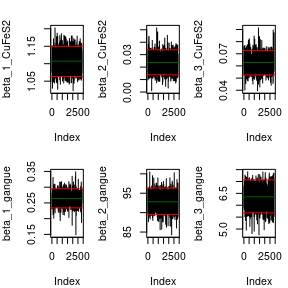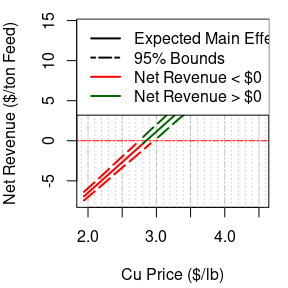Two_Node_Process

2020-11-09

library(BayesMassBal)

The function BMB is used with a two node process and simulated data.The constraints around these process nodes are:

\begin{align} y_1 &= y_2 +y_4\\ y_2 &= y_3 +y_5 \end{align}

Therefore the matrix of constraints, C is:

C <- matrix(c(1,-1,0,-1,0,0,1,-1,0,-1), nrow = 2, ncol = 5, byrow = TRUE)
C
#>      [,1] [,2] [,3] [,4] [,5]
#> [1,]    1   -1    0   -1    0
#> [2,]    0    1   -1    0   -1

The constrainProcess function in the BayesMassBal package is used to generate an X matrix based on C that will later be used with the BMB function.

X <- constrainProcess(C = C)
X
#>      [,1] [,2] [,3]
#> [1,]    1    1    1
#> [2,]    1    0    1
#> [3,]    1    0    0
#> [4,]    0    1    0
#> [5,]    0    0    1

Constraints can also be imported from a .csv file. The path to a file, included in the BayesMassBal package, for this process can be found and constraints can be imported by specifying the location for the file argument for constrainProcess as shown below:

constraint_file_location <- system.file("extdata", "twonode_constraints.csv",package = "BayesMassBal")
X <- constrainProcess(file = constraint_file_location)

The previously simulated data is loaded from a .csv file using the importObservations() function. The local location of the the file imported below can be found by typing system.file("extdata", "twonode_example.csv",package = "BayesMassBal"). View the document in Excel to see how your data should be formatted for import. Note: it is not required that the entries into the *.csv file are separated by ";".

y <- importObservations(file = system.file("extdata", "twonode_example.csv",
package = "BayesMassBal"),
header = TRUE, csv.params = list(sep = ";"))

Then, the BMB function is used to generate the distribution of constrained masses from the data with cov.structure = "indep".

indep.samples <- BMB(X = X, y = y, cov.structure = "indep", BTE = c(100,3000,1), lml = TRUE, verb = 0)

The output of BMB is a BayesMassBal object. Special instructions are designated when feeding a BayesMassBal object to the plot() function. Adding the argument layout = "dens" and indicating the mass balanced flow rate for CuFeS2 at $$y_3$$ should be plotted using a list supplied to sample.params, the desired distribution can be plotted with its 95% Highest Posterior Density Interval.

plot(indep.samples,sample.params = list(ybal = list(CuFeS2 = 3)),
layout = "dens",hdi.params = c(1,0.95))It is also possible to generate trace plots to inspect convergence of the Gibbs sampler. Here are trace plots for $$\beta$$

plot(indep.samples,sample.params = list(beta = list(CuFeS2 = 1:3, gangue = 1:3)),layout = "trace",hdi.params = c(1,0.95))A quantitative diagnostics for convergence and autocorrelation are available as part of the output from BMB:

indep.samples$diagnostics #>$beta
#> $beta$CuFeS2
#>   index        cd      ess
#> 1     1 -1.230771 2427.845
#> 2     2  1.928846 2900.000
#> 3     3 -0.937918 3609.719
#>
#> $beta$gangue
#>   index         cd      ess
#> 1     1  0.3662101 2566.943
#> 2     2 -2.4501016 2900.000
#> 3     3  0.0505272 1949.028
#>
#>
#> $Sig #>$Sig[]
#>   index         cd      ess
#> 1     1 -0.7203073 2568.652
#> 2     2  0.1874695 3355.865
#> 3     3  0.4034246 2935.132
#> 4     4 -0.6040698 2216.813
#> 5     5  1.9407674 1945.730
#>
#> $Sig[] #> index cd ess #> 1 1 -0.1866999 2904.780 #> 2 2 0.7179901 2497.712 #> 3 3 -0.1567601 2212.362 #> 4 4 -0.1889470 2620.413 #> 5 5 0.2429657 2128.884 The model with independent variances may not be the best fitting model. Models specifying covariance between sample locations for a single component, and covariance between components at a single location are fit. component.samples <- BMB(X = X, y = y, cov.structure = "component", BTE = c(100,3000,1), lml = TRUE, verb = 0) location.samples <- BMB(X = X, y = y, cov.structure = "location", BTE = c(100,3000,1), lml = TRUE, verb = 0) Computing $$\log(\mathrm{Bayes Factor})$$ for $$BF = p(y|\texttt{indep})/p(y|\texttt{component})$$: indep.samples$lml - component.samples$lml #>  -126.913 Then comparing $$p(y|\texttt{component})$$ to $$p(y|\texttt{location})$$ component.samples$lml - location.samples$lml #>  0.8313626 Shows there is little difference between the models where cov.structure = "location" and cov.structure = "component", but both of these models better explain the data than cov.structure = "indep". We can view a summary of the favored model by passing a BayesMassBal object to the summary function. While not done in this case, the summary table can be saved by passing the desired name of a *.csv file to the export argument. summary(component.samples, export = NA) #> Mass Flow Rates: #> #> CuFeS2: #> -------------------- #> Sampling Location Expected Value 95% LB 95% UB #> 1 1.19860888 1.14504173 1.24894010 #> 2 1.17339882 1.12748652 1.23334472 #> 3 1.11056483 1.05948452 1.16831064 #> 4 0.02521007 0.01694020 0.03434161 #> 5 0.06283399 0.05187997 0.07341624 #> #> gangue: #> -------------------- #> Sampling Location Expected Value 95% LB 95% UB #> 1 100.2687657 95.6808511 104.9860650 #> 2 6.6631495 5.8638558 7.5120136 #> 3 0.2603553 0.2270064 0.2932927 #> 4 93.6056162 89.3126606 98.1107686 #> 5 6.4027942 5.5672713 7.1908586 #> #> Total: #> -------------------- #> Sampling Location Expected Value 95% LB 95% UB #> 1 101.467375 96.776984 106.092720 #> 2 7.836548 7.045612 8.709765 #> 3 1.370920 1.308823 1.433180 #> 4 93.630826 89.341476 98.134499 #> 5 6.465628 5.664312 7.287156 #> #> #> log-marginal likelihood: #> -66.0464453368283 The main effect of a variable independent of the process can be calculated by supplying a function, fn that takes the arguments of mass balanced flow rates ybal, and the random independent and uniformly distributed variables x. Information can be gained on the main effect of a particular element of x, xj, on fn using the mainEff function. Output from mainEff includes information on the distribution of $$E_x\lbrack f(x,y_{\mathrm{bal}})|x_j \rbrack$$. fn_example <- function(X,ybal){ cu.frac <- 63.546/183.5 feed.mass <- ybal$CuFeS2 + ybal$gangue # Concentrate mass per ton feed con.mass <- (ybal$CuFeS2 + ybal$gangue)/feed.mass # Copper mass per ton feed cu.mass <- (ybal$CuFeS2*cu.frac)/feed.mass
gam <- c(-1,-1/feed.mass,cu.mass,-con.mass,-cu.mass,-con.mass)
f <- X %*% gam
return(f)
}

rangex <- matrix(c(4.00 ,6.25,1125,1875,3880,9080,20,60,96,208,20.0,62.5),
ncol = 6, nrow = 2)
mE_example <- mainEff(indep.samples, fn = "fn_example",rangex =  rangex,xj = 3, N = 25, res = 25)

A plot of the output can be made. To get lines that are better connected, change increase N in the mainEff function.

m.sens<- mE_example$fn.out[2,] hpd.sens <- mE_example$fn.out[c(1,3),]
row.names(hpd.sens) <- c("upper", "lower")
g.plot <- mE_example$g/2000 y.lim <- range(hpd.sens) lzero.bound <- apply(hpd.sens,1,function(X){which(X <= 0)}) lzero.mean <- which(m.sens <= 0) main.grid <- pretty(g.plot) minor.grid <- pretty(g.plot,25) minor.grid <- minor.grid[-which(minor.grid %in% main.grid)] y.main <- pretty(hpd.sens) opar <- par(no.readonly =TRUE) par(mar = c(4.2,4,1,1)) plot(g.plot,m.sens, type = "n", xlim = range(g.plot), ylim = y.lim, ylab = "Net Revenue ($/ton Feed)", xlab=  "Cu Price ($/lb)") abline(v = main.grid, lty = 6, col = "grey", lwd = 1) abline(v = minor.grid, lty =3, col = "grey", lwd = 0.75) abline(h = 0, col = "red", lwd = 1, lty = 6) lines(g.plot[lzero.mean],m.sens[lzero.mean],col = "red", lwd =2) lines(g.plot[-lzero.mean[-length(lzero.mean)]],m.sens[-lzero.mean[-length(lzero.mean)]],col = "darkgreen", lwd =2) lines(g.plot[lzero.bound$lower],hpd.sens[2,][lzero.bound$lower], lty = 5, lwd = 2, col = "red") lines(g.plot[-lzero.bound$lower],hpd.sens[2,][-lzero.bound$lower], lty = 5, lwd = 2, col = "darkgreen") lines(g.plot[lzero.bound$upper],hpd.sens[1,][lzero.bound$upper], lty = 5, lwd = 2, col = "red") lines(g.plot[-lzero.bound$upper],hpd.sens[1,][-lzero.bound$upper], lty = 5, lwd = 2, col= "darkgreen") legend("topleft", legend = c("Expected Main Effect", "95% Bounds", "Net Revenue <$0", "Net Revenue > \$0"), col = c("black","black","red", "darkgreen"), lty = c(1,6,1,1), lwd = c(2,2,2,2), bg = "white")par(opar)